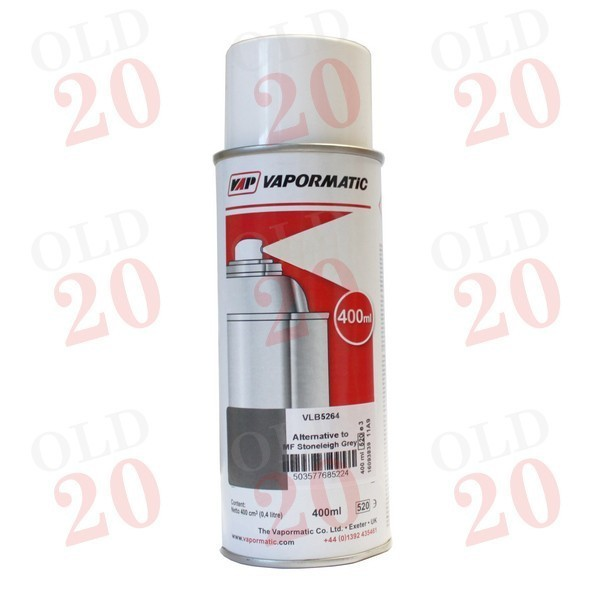View larger

# Paint - MF Stoneleigh Grey (400ml)

7330005A

New

£ 15.30Tractors < Massey Ferguson < Pre-100 Series < 25
Tractors < Massey Ferguson < Pre-100 Series < 30
Tractors < Massey Ferguson < Pre-100 Series < 35
Tractors < Massey Ferguson < Pre-100 Series < 35X
Tractors < Massey Ferguson < Pre-100 Series < 65
Tractors < Massey Ferguson < Pre-100 Series < 65 MKII
Tractors < Massey Ferguson < 100 < 122
Tractors < Massey Ferguson < 100 < 130
Tractors < Massey Ferguson < 100 < 133
Tractors < Massey Ferguson < 100 < 134C
Tractors < Massey Ferguson < 100 < 135
Tractors < Massey Ferguson < 100 < 134V
Tractors < Massey Ferguson < 100 < 135V
Tractors < Massey Ferguson < 100 < 140
Tractors < Massey Ferguson < 100 < 145
Tractors < Massey Ferguson < 100 < 145MKIII
Tractors < Massey Ferguson < 100 < 145S
Tractors < Massey Ferguson < 100 < 145V
Tractors < Massey Ferguson < 100 < 148
Tractors < Massey Ferguson < 100 < 148MKIII
Tractors < Massey Ferguson < 100 < 150
Tractors < Massey Ferguson < 100 < 152
Tractors < Massey Ferguson < 100 < 152F
Tractors < Massey Ferguson < 100 < 152MKIII
Tractors < Massey Ferguson < 100 < 152S
Tractors < Massey Ferguson < 100 < 152V
Tractors < Massey Ferguson < 100 < 154
Tractors < Massey Ferguson < 100 < 154C
Tractors < Massey Ferguson < 100 < 154F
Tractors < Massey Ferguson < 100 < 154S
Tractors < Massey Ferguson < 100 < 154V
Tractors < Massey Ferguson < 100 < 155
Tractors < Massey Ferguson < 100 < 158
Tractors < Massey Ferguson < 100 < 158F
Tractors < Massey Ferguson < 100 < 158 MKIII
Tractors < Massey Ferguson < 100 < 158S
Tractors < Massey Ferguson < 100 < 158V
Tractors < Massey Ferguson < 100 < 164TF
Tractors < Massey Ferguson < 100 < 164TS
Tractors < Massey Ferguson < 100 < 164TV
Tractors < Massey Ferguson < 100 < 165
Tractors < Massey Ferguson < 100 < 165 MKII
Tractors < Massey Ferguson < 100 < 168
Tractors < Massey Ferguson < 100 < 168F
Tractors < Massey Ferguson < 100 < 168 MKIII
Tractors < Massey Ferguson < 100 < 168S
Tractors < Massey Ferguson < 100 < 174C
Tractors < Massey Ferguson < 100 < 174F
Tractors < Massey Ferguson < 100 < 174S
Tractors < Massey Ferguson < 100 < 174V
Tractors < Massey Ferguson < 100 < 175
Tractors < Massey Ferguson < 100 < 175S
Tractors < Massey Ferguson < 100 < 178
Tractors < Massey Ferguson < 100 < 185
Tractors < Massey Ferguson < 100 < 184
Tractors < Massey Ferguson < Accessories < Paint

Supplier Reference :

 Section Accessories Tractor Massey Ferguson Colour Grey
Size: 400ml Aerosol

£ 7.80